Games
Problems
Go Pro!

# Algebra

Reference > Mathematics > Algebra

# Featured ReferenceHow to simplify various kinds of radical expressions, including square roots, cube roots, and expressions with radicals in the denominators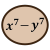Learn more about polynomials and polynomial equations. How to find solutions, understanding conjugates, relation between roots and coefficients, and t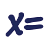Solving systems of equations, including systems of two and three unknowns, as well as fractional equations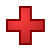A unit on rational expressions, including adding, subtracting, multiplying, dividing algebraic fractions, as well as solving fractional equations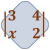A brief unit on calculation, reduction, and uses of determinantsSome tips and tricks for factoring cubic polynomials and other higher degree polynomials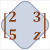Understanding what a matrix is, and different ways of manipulating matricesFundamental counting principle, permutations, combinations, and distinguishable permutations

# Full Directory Listing

Algebraic Fractions, Basic Word Problems, Cat and Mouse Variation, Complex Numbers, Counting Principles, Determinants, Factoring, Factoring Higher Degree Polynomials, Matrices, Polynomials, Sequences and Series, Simplifying Radicals, Systems of Equations, The Quadratic Formula# Blogs on This SiteReviews and book lists - books we love!The site administrator fields questions from visitors.Like us on Facebook to get updates about new resources